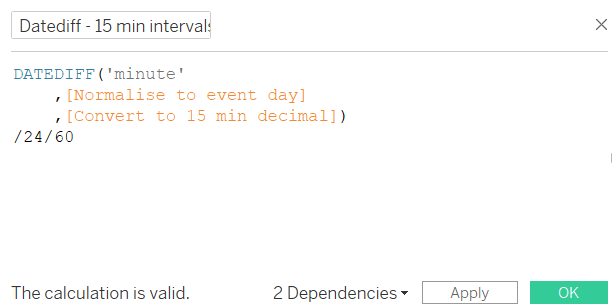# Tableau how to display 15 minute intervals

//

This article explains how to report data in different time intervals in Tableau. For example, to show 15 minute intervals, split every hour into quarters; 00:15, 00:30, …, 23:30, 23:45.

This is useful if you want to use Tableau to spot trends in outages, or other similar time-based situations.

To segment in 5 minute, 10 minute or 30 minute intervals, jump to the bottom of this article to learn how to create custom time intervals in Tableau.

Consider the following time and date data:

The goal is to display this data in 15 minute intervals, so, for example, every event between 19:15 and 19:30 is grouped, 19:30 to 19:45 in a different group, etc, regardless of the date of the event.

## Truncate the data to the nearest 15 minute interval

To round the data to 15 minute buckets, use a calculated field. This converts the date time to a decimal, then converts it back to a date time. Unfortunately, more straightforward date functions, such as the DATETRUNC function, don’t work for more complex time periods.

This formula truncates to the 15 minute interval:

### Copy the code to truncate to 15 mins

`[Convert to 15 min decimal]`

`DATETIME((INT(FLOAT([Event Date Time])*96))/96)`

This keeps the date within the data, which we don’t want for this exercise. We’re only interested in the time component.

Regardless of the actual date, we want to only display the hours and minutes of that date. Therefore normalise the time, removing the date component.

To normalise the time, find the number of minutes between the 15 minute interval and midnight.

Firstly, use a simple DATETRUNC formula to set each event date to midnight:

### Copy the code to truncate each day to midnight

`[Normalise to event day]`

`DATETRUNC('day',[Event Date Time])`

Next, calculate the difference in minutes between these fields. To do that, use a DATEDIFF function.

`[Diff in Minutes]`

`DATEDIFF('minute',[Normalise to event day],[Convert to 15 min decimal])`

To report this in 15 minute time buckets, there’s one final step. To display hh:mm in Tableau, convert the number of minutes to a decimal. Modify the DATEDIFF calculation to:Calculates the number of minutes between midnight and the 15-min truncated event time – then convert to decimal

### Copy the code to create the 15 minute intervals as decimal

`[15 Min Intervals Decimal]`

`DATEDIFF('minute',[Normalise to event day],[Convert to 15 min decimal])/24/60`

This field is numeric rather than datetime. Use a custom format of hh:nn to display hour:minutes based on a 24 hour clock.

Lay the calculations out in a table to see the results:

Now the 15 min interval calculations are good, use in a simple bar chart to show the distribution in 15 minute intervals. Click the image below to see and download from Tableau Public.

## How to show 5, 10 or 30 minute Time intervals in Tableau

Changing the interval length is simple mathematics. Remember the 15 minute interval truncation formula at the beginning of this post:

`DATETIME((INT(FLOAT([Event Date Time])*96))/96)`

The number 96 is key to this; understanding the source of that number will open up other custom time truncation.

To truncate time, you need to know how many intervals there are per hour, then multiply it by 24 hours. For example, there are 4 x 15 minute intervals per hour. 4 x 24 = 96.

Therefore, to segment in 30 minute intervals, there are 2 x 20 min intervals per hour. 2 x 24 = 48, so the formula changes to:

`DATETIME((INT(FLOAT([Event Date Time])*48))/48)`

To segment using 10 minute intervals, change the number to 6 x 24 = 144

`DATETIME((INT(FLOAT([Event Date Time])*144))/144)`

For 5 minute intervals, use 12 x 24 = 288